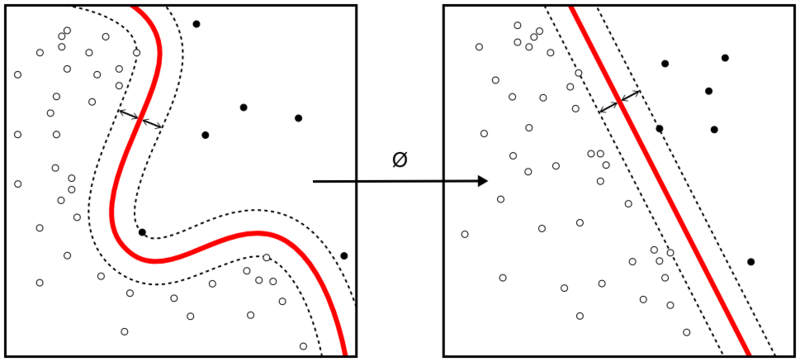## How to program the Support Vector Machines algorithm

Support Vector Machine is one of the most commonly used supervised machine learning algorithms for data classification. A binary classifier, the support vector machine algorithm works in vector space to sort data points by finding the best hyperplane separating them into two groups. Thanks to its reliance upon vectors, it finds frontiers between groups of data points even in nonlinear patterns and features spaces of high dimensions.Continue reading “How to program the Support Vector Machines algorithm”

## Decision boundaries

Separating positive and negative example with a straight line that is as far as possible from both positive and negative examples, a median that maximizes the space between positive and negative examples.

Constraints are applied to build a support vector (u) and define a constant b that allow to sort positive examples from negative ones. The width of a “street” between the positive and negative values is maximized.

Going through the algebra, the resulting equation show that the optimization depends only on the dot product of pair of samples.

The decision rule that defines if a sample is positive or negative only depends on the dot product of the sample vector and the unknown vector.

### No local maximum

Such support vector algorithm can be proven to be evolving in a convex space, meaning that it will never be blocked at a local maximum.

### Non linearity

The algorithm cannot find a median between data which cannot be linearly separable. A transformation can however be applied to the space to reorganize the samples so that they can be linearly separable. Certain transformations can however create an over fitting model that becomes useless by only sorting the example data.